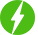# Vedic Maths

1

Vedic Maths, Vedic Maths : Tricks For Faster Calculation.

## Course Description

Vedic Maths Tricks for Faster Calculation.

Learn Tricks for Faster Addition , Subtraction , Multiplication and Division.

Completing the Whole

Splitting

Subtraction

All from Nine Last from Ten

Splitting

Vinculum

Multiplication

Vertically and Cross-wise method

Base Method

One More than the previous

Multiplication with 11,12 and 99.

Division

Nikihilam Method.

Division by 9 and 99.

See some interesting observation on Square , Cube , Square roots and Cube roots.

Vedic Mathematics is a collection of Techniques/Sutras to solve mathematical arithmetic in easy and faster way. It consists of 16 Sutras (Formulae) and 13 sub sutras (Sub Formulae) which can be used for problems involved in arithmetic, algebra, geometry, calculus, conics.

Vedic Mathematics is a system of mathematics which was discovered by Indian mathematician Jagadguru Shri Bharathi Krishna Tirthaji in the period between A.D. 1911 and 1918 and published his findings in a Vedic Mathematics Book by Tirthaji Maharaj.

Contrary to common belief, Vedic Mathematics is not an alternate numeral system, or even methodology. It is a set of mathematical rules formulated to supplement the usual methods of calculations. Vedic Mathematical Sūtras provide simple, straight forward alternates to calculate, the otherwise cumbersome and complicated calculations.

Vedic Mathematics is a unique contribution of Ancient India to the world, particularly to the world mathematics often termed as ‘tricks’ or ‘mental maths ‘ by the western world which, over thousands of years, remains the oldest and the fastest method for calculations.

We will be happy to hear your thoughtsRegister New Account
• Total (0)
0